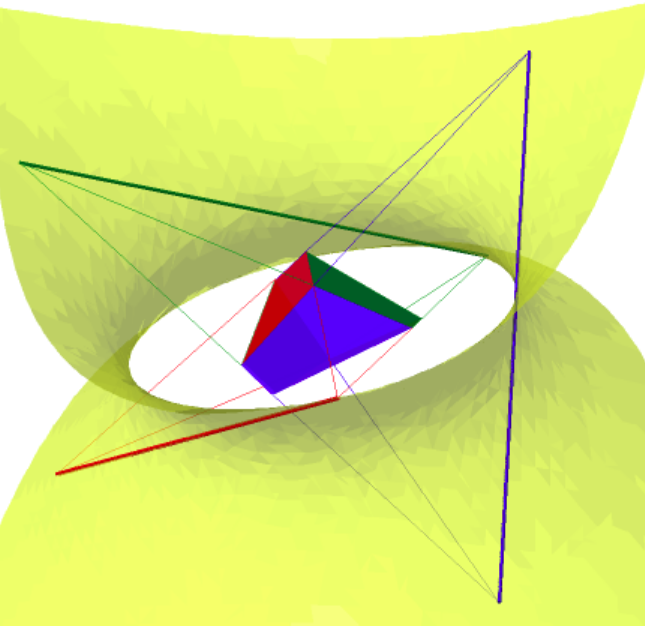### Highlights#### Projective geometry of Wachspress coordinates

ICERM - April 2019The barycentric coordinates of a triangle were introduced by August F. Möbius in 1827. They describe a point in a triangle relative to the three vertices of the triangle. A point in the interior of a triangle splits the triangle into three smaller triangles, as seen in the first figure. The ratios of the three areas of the smaller triangles by the area of the big triangle are the barycentric coordinates of that point. For instance, in the first figure, the area of the red triangle is ½ of the area of the big triangle. The areas of the blue and the green triangle are each ¼ of the area of the big triangle.

In 1975 Eugene L. Wachspress described a generalized version of barycentric coordinates for other polygons in the plane, such as quadrangles, pentagons, hexagons, etc. In 1996 Joe Warren extended this generalization to higher dimensions, namely to polytopes in dimensions three and higher. There exist other generalizations of barycentric coordinates to higher dimensional polytopes (e.g. mean value coordinates), so the constructions by Wachspress and Warren are commonly known as Wachspress coordinates. These coordinates and other generalized barycentric coordinates have many applications, such as mesh parameterizations in geometric modelling, deformations in computer graphics, or polyhedral finite element methods. A nice overview on different versions of barycentric coordinates, their history and their applications is [Flo15].

Wachspress and Warren used different ideas and techniques to construct their Wachspress coordinates.  To bridge both approaches, Kathlén Kohn and Kristian Ranestad developed a proof for the following conjecture: For every polytope (whose defining hyperplane arrangement is simple) there is a unique polynomial equation of minimal degree which is zero along the non-faces of the polytope [KK19]. For instance, a perturbed cube has three non-faces: There are three pairs of opposite sides on the cube (red, green and blue in the second figure), and each pair of planes spanned by two opposite sides on the cube intersects in a line (also in red, green and blue, respectively). So the three non-faces of the perturbed cube are three lines. The conjecture proven by Kohn and Ranestad states in this special case the well known fact that there is a unique quadratic polynomial whose zero set contains the three lines. This quadratic zero set is visualized in yellow in the second figure.

In addition to the applications of barycentric coordinates mentioned above, this result is relevant in statistics (when studying probability distributions on polytopes) and it relates to the classification of algebraic varieties with a unique canonical divisor, a rich and classical topic in algebraic geometry.

The Fall 2018 program on Nonlinear Algebra at ICERM had many activities and yielded many more new results in algebraic geometry and its applications, such as computational methods, optimization, graphical models in statistics, matrix completion or computer vision.

REFERENCES:
[Flo15] M. S. Floater: Generalized barycentric coordinates and applications, Acta Numerica 24 (2015), 161-214.
[KK19] K. Kohn and K. Ranestad: Projective geometry of Wachspress coordinates, preprint available at https://arxiv.org/pdf/1904.02123.pdf (2019).